## Thursday, October 30, 2008

### K-Center and Affinity Propagation Clustering

Recent release of VisuMap implements a new clustering algorithm called Affinity Propagation (AP). AP provides similar service as the metric sampling (MS) algorithm that has been available in VisuMap for a while. In this blog I will compare AP with MS from practical point of view with concrete samples.

First, a few words about the implementations of these algorithms. MS is basically a variation of the k-mean algorithm that uses the expectation maximization (EM) method to solve the k-medoid problem. Such algorithms are sometimes called k-center or k-medoid algorithm. Simple direct implementation of k-medoid algorithm often just find bad solutions (i.e. local minimums). People normally has to repeat the algorithm many many times to find an acceptable solution. The MS algorithm implemented in VisuMap uses a stochastic mechanism to avoid or reduce such local minimum problem.

AP uses message passing mechanism to find representative exemplars within dataset with a similarity structure. AP is basically a deterministic algorithm, it does not require random initialization or randomness during the optimization process. However, AP algorithm often suffers from the oscillation problem in the way that the algorithm wanders around several local minimums for quite long time. To reduce the oscillation, we can use a large dumping factor as suggested in the original paper of AP. But a more efficient way seems to be using small randomness when assigning preferences to the data points. By default, the implementation of AP in VisuMap enables this randomness.

Thus, both MS and AP in VisuMap are of stochasitc nature. I have applied the two algorithms each 500 times on the dataset SP500 (each data point contains the weekly price history of a stock in a year) and got all together 1000 different clustering solutions (each solution is a selection of 25 exemplar points). Using the t-SNE algorithm I created the map of these 1000 solutions as shown in the following picture: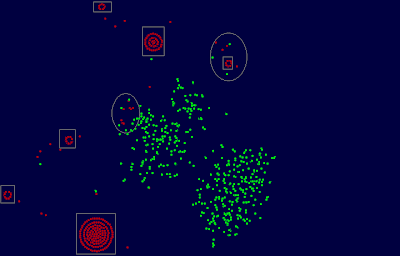In above map each red/green dot represents a solution found by AP/MS algorithm respectively. As a special characteristic of t-SNE algorithm, identical solutions will be arranged as one more circles. That means, dots in a rectangle in above map represent acutally identical solutions.

We notice first that the two algorithms found very different solutions as red and green dots are clearly separated from each other. There are only two small regions (marked by ovals) where the two algorithms found similar solutions. Secondly, we notice that MS found a large number of different solutions. AP found just few different solutions. This means that the AP optimization process often converged to common solutions.

The following table shows the statistics about the solutions found by AP and MS:

 MetricSampling AffinityPropagation Min Error 0.4972 0.4901 Max Error 0.5058 0.5035 Average Error 0.5004 0.4960 Process Timein Seconds 5 4

In the above table the error of a solution is the average distance of the data points to their cluster center (i.e. exemplars). The average error is calculated over the 500 solutions found by each of the two algorithms. We can see that AP has found, on the average, better solutions than MS, but the improvement is only about 0.4%. So, it is fair to say that these two algorithms performed similarly with respect to this dataset.

The situation changed a lot when I applied the two algorithms to a different dataset, namely the dataset PharMine171 discussed in previous blog entry. This dataset contains about 1000 binary 171-dimensional vectors , whereas the SP500 dataset contains 500 40-dimensional vectors with real values. The following is the map of the clustering solutions and the corresponding statistics.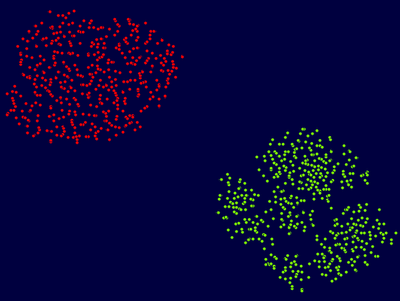MetricSampling AffinityPropagation Min Error 2.1775 2.1101 Max Error 2.2321 2.1686 Average Error 2.1972 2.1603 Process Timein Seconds 6 30

From above map we can see that AP and MS still produced different set of solutions. AP also produced more different solutions than with the previous dataset. This means that, with respect to this dataset, AP is more sensitive to small variations/noise in the input data. This phenomenon might be explained as following: Because this dataset contains binary vectors, many similarities between data points might have exact equal values. Those equivalence of similarity will be broken different by small variations in the input data. Since AP indeed uses operation like max() to distinguish even infinitesimal differences, small variations may lead to significantly different solutions.

We also notice that AP is substantially slower than MS. For larger datasets, the performance difference will be even larger. For instance, for a dataset with about 4000 data points, MS is more than 100 times faster than AP. It should be pointed that both implementations of AP and MS uses the full similarity matrix. It remains to see how much speedup can be achieved by using sparse matrix.

More interestingly we see that the solutions found by AP is about 3.7% better than those found by MS; and even the worst solution found by AP is better than the best solution found by MS.

Now, one would ask the question: Does AP always find better solutions than MS? Unfortunately, this is not the case. The following picture shows the 3D presentation of the spheroid dataset that contains 1825 data points forming together a spheroid: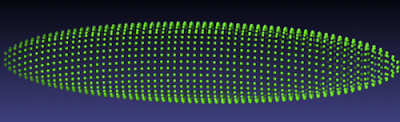As be shown in the following test statistics, solutions found by MS is about 3.7% better than those found by AP:

 MetricSampling AffinityPropagation Min Error 5.9029 5.9029 Max Error 6.4034 6.6483 Average Error 6.0948 6.3199 Process Timein Seconds 9 284

Conclusions:
I believe AP is a useful and promising addition to the arsenal of clustering algorithms. Not only that AP can find better solutions, it also finds different solutions as existing algorithms. As we know that there are many different clustering problems. AP doesn't seem to be designed to directly attack the k-medoid problem, but nevertheless it is competitive to those algorithms directly targeting the k-medoid problem. This indicates that AP may have potential to attack a much larger class of clustering problems.

## Tuesday, October 21, 2008

### Clustering clusters

Many clustering algorithms, like k-mean algorithm, are of stochastic nature in the sense that they produce different partitions (of a data set) depending on the random initialization. In this blog I am going to use the mapping service provided by VisuMap to investigate the partitions generated by a particular clustering algorithm. In other word, I want to visualize the clusters among partitions generated by a clustering algorithm.

The clustering algorithm I am going to look at is the metrical sampling (MS) clustering algorithm which is basically a k-mean algorithm with some randomization extension. For a given set of data points with a distance matrix, MS algorithm tries to find a set of sample points, so that minimizes the average error, that is average distance of the data points to their nearest sample point (also called exemplar). Thus, MS algorithm is one of many available algorithms to solve the so called k-medoid clustering problem (which is an NP complete problem according to certain literature).

For a dataset of N data points, MS algorithm produces a N dimensional binary vectors. Each component is a binary value that decides whether a data point is selected as a sample point. So, when we run the MS algorithm many times with different random initializations, we will get a list of N dimensional binary vectors. I am interested to see clusters or patterns among those binary vectors. For this study, I picked up the dataset PharMine171 that contains 1025 fingerprints of 171 pharmacologically interesting compounds. I have run MS algorithm on the dataset for 1000 times. The following is a map of the 1000 partitions ( produced with the t-SNE mapping algorithm):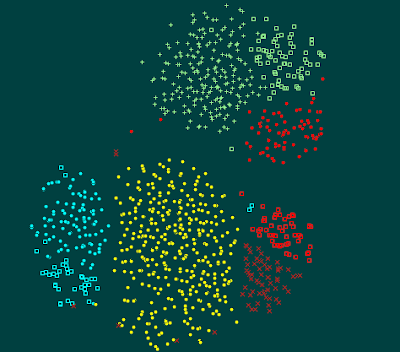In above map, each spot represents a partition generated by one run of MS algorithm. Closely located partitions means they share more sample points. The coloring is done manually to highlight the clusters. We can easily see that there are 8 or 9 clusters among the 1000 partitions.

Now, one question that raises naturally is: Do the partitions in one cluster have similar qualities (i.e. average error)? In order to answer this questions I opened a bar view window that displays the average error of each partition in an ordered bar view as follows: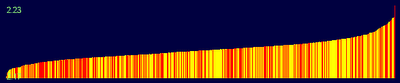In above picture, each bar (in yellow or red color) represents a partition. The height of the bar indicates their average error. The red bars represent those partitions selected manually on the partition maps. So, when we select a cluster in the partition map, we can easily see the corresponding distribution of average error of that clusters. The following two pictures show two such distributions: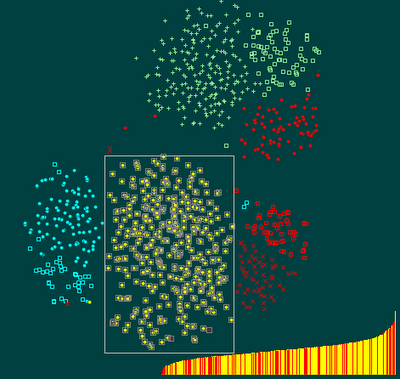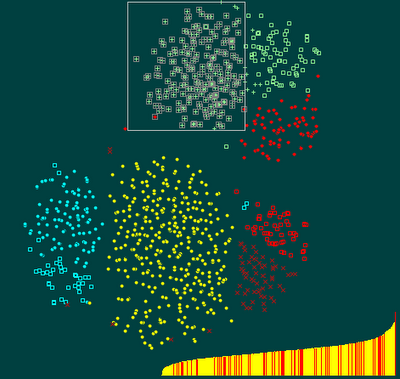We notice that the average error in a cluster is spread all over the whole error range (from very bad to very good). This means, similar partitions can have very different qualities. Thus, the answer to above questions is negative.

More in general, I suppose this study indicates that any algorithm trying to find good solutions for the k-medoid problem need a mechanism to allow global variations in the searching space. The MS algorithm implemented in VisuMap does this through controlled randomness. Another interesting algorithm, named affinity propagation seems to do that with help of 2N2 addition system states (called messages). Affinity propagation is also supported in VisuMap. I will do some comparison between MS and AP in another blog.

## Saturday, September 13, 2008

### Feature selection by clustering

I have blogged before that there is a "symmetry" between data clustering and dimensionality reduction: whereas the former reduces the number of rows; the latter reduces the number of columns in a data table. Thus, we can theoretically convert any clustering algorithm to a dimensionality reduction method by transposing the data table, and vise verse.

In this blog I am going to demonstrate how to use the metric sampling algorithm to reduce dimensionality with a concrete dataset. My sample dataset (PharMine171) contains data for 171 pharmacologically interesting compounds. Each data point contains 1025 binary vectors which indicate whether the compound contains particular fragments (ie. molecular sub-structurs). Thus, the dataset is a 171 x 1025 table with binary values and its dimension is 1025.

In order the reduce the dimensionality we first transpose the table to get a 171 dimensional dataset with 1025 data points (each data point now represents a fragment). In order to get feeling about the transposed dataset I have created 2 dimensional map for it (with VisuMap) as be shown in the following picture:Map of 1025 binary features

Above map shows clearly some clusters among the 1025 data points. In order to find representative columns in our original dataset, we need to find representative rows in the new data table. In order to do so, we apply the metric sampling algorithm (available in VisuMap) that selects representative subset of data points. I have configured the algorithm to select 25 data points. The following picture shows how the 25 data points are distributed among the 1025 data points: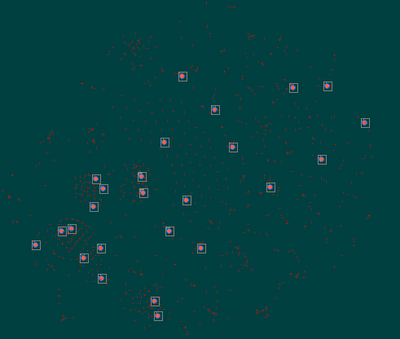We can then export the 25 data points as a 25 x 171 table, and transpose the table to get an 171 x 25 data table. This table is then our new dataset with reduced dimension.

Now, how can we tell that the reduced dataset approaches the original dataset? We can do this pretty easily with VisuMap by creating maps for both datasets. If the two datasets contain about the same information, their maps should be visually similar to each other. The following picture shows the map of the original dataset (on the left side) and of the reduced dataset (on the right side). They are both created with the SMACOF mapping method. We see that the map of the reduced dataset reveals clearly the three clusters as the original dataset, but it shows less details within the clusters.Notice that the metric sampling algorithm implemented in VisuMap is a variation of the widely known k-medoid algorithm. The recent release of VisuMap (version 2.6.807) utilized a randomization strategy in the optimization process that made the algorithm much more robust and less prone to local minimum problem; which has been a big issue for the k-medoid algorithm.

## Thursday, September 4, 2008

### On similarity metrics for chemical compounds

Recently, Yap Chun Wei has posted a dataset on the pharmine blog. The dataset consists of fingerprints of 171 pharmacologically interesting compounds. Just to recapture, the fingerprint of a compound is here a vector of 1025 binary flags, each flag in the vector indicates whether a particular molecular fragment is present in the compound. There are many ways to calculate fingerprints. Depending on the nature of the problem, you can use different algorithms or different collection of fragments. The mentioned dataset, for instance, used OpenBabel to calculate those fingerprints.

The dataset constains 3 different groups of compounds: penicillins, cephalosporins, fluoroquinolones. Using VisuMap Yap created different maps of these 171 compounds which showed, more or less, the cluster structure of the dataset. I personally find that the PCA map provides the best visualization. The following picture is a PCA map I created with VisuMap for this dataset:

In above map, the 3 compound groups are displayed as glyphs in 3 different colors. The coloring are done manually with VisuMap based on known information about these groups. Although, for this dataset, you can get almost exactly these 3 clusters using the k-mean algorithm provided by VisuMap. The bar diagram in the picture shows the presence frequency of the 1025 fragments among the 171 selected compounds. That means, a higher bar indicates that a particular fragment is present in more compounds.

The above map visualizes the similarity information between the 171 compounds. That means, closely located compounds will have similar fragment collections and therefore similar pharmacological properties. The similarity information are basically encoded in the fingerprints. Thus, the method to calculate of those fingerprints is naturally critical for this kind of data analysis.

In order to better understand those fingerprints we can created a map of those 1025 features with VisuMap. In order to do so, we simply transpose the binary data table (via the menu Edit>Filter Data>Transpose Table in VisuMap), so that each binary feature becomes a new data point; and each compound become a feature in the transposed dataset. We can then pick a mapping algorithm and metrics to create a feature map. The following picture shows such a map created with the t-SNE algorithm and the tanimoto dissimilarity metric:

Above picture shows 4 or 5 clear clusters on the left side represented by colored glyphs. The rest are more or less randomly distributed. It turned out that those yellow-square features are those fragments which are NOT present in any of those 171 compounds (all bits are zero). Therefore, they carry no direct information about our compounds. Interestingly, these zero vectors form together a homogeneous cluster in the map.

Other clusters in above map represent groups of fragments which have high frequency and are informative to distinguish the three compound groups in the original dataset. We can verify this with the help of the bar diagram in VisuMap as follows:

We first open the feature map in a separate window (via the menu Tools>Map Snapsot). Then open the compound map, and then select all compounds and open the bar view through the context menu "Bar View". The bar view by default displays the frequency in the order as given in the transposed data table. We then sort the bars through the context menu "Sort Values" so that bars are displayed in the order from low frequency to high frequency as depicted in the following two picture:The sorted bar view shows 3 plateaus which correspond to clusters in the feature map and in the compound maps. In order to see the correspondence we select a plateau in the bar view with the mouse, the snapshot window of the feature map will automatically high light those selected features. As we can see in the following picture, the selected plateau of features clearly correspond to a particular cluster in the feature map (the marked cluster at lower left corner).

We notice that some of the clusters in the feature map show some fine sequential structure that may lead to more hints about the internal structure of the fragment collections.

With the knowledge about informative feature clusters we can, for instance, reduce the number of features significantly without significant loss of information about the clusters in the original dataset. The VisuMap dataset folder PharMine171.xvmz (zipped XML file) includes a reduced dataset with 298 features that characterizes similar similarity information as the original dataset with 1025 features.

The above VisuMap dataset folder also contains feature maps created with other mapping algorithms. It is interesting to notice that for the feature map dataset, the t-SNE algorithm provides the best visualization, whereas the result of the PCA algorithm is rather disappointing.

## Wednesday, August 13, 2008

### New mapping algorithm t-SNE

Recently I spent some time to experiment with the t-SNE mapping algorithm proposed by L. Maaten and G. Hinton in Visualizing Data Using t-SNE. The algorithm is a variation of the SNE algorithm developed few years ago.

The t-SNE home site provides source code, technical papers and many samples, so that it is pretty easy to test the algorithm. The matlab source code is provided as a component in a matlab toolbox that also implements many other popular methods for dimensionality reduction. The web site also provides a binary executable that is optimized for the Intel/Windows platform. It took me a while to figure out its binary data format, but the time spent is worthwhile, as it runs much faster than the matlab version.

As demonstrated in its technical paper, t-SNE has the nice capability to show the cluster structure of high dimensional datasets in low dimensional maps. More interestingly, I noticed that t-SNE is able to segment dataset with complex structure in a natural way to preserve relevant information from certain perspective. For instance, the left map in the following picture shows a dataset that forms two crossing squares in 3D space, the map on the right side is a 2D map created with t-SNE. We notice that t-SNE segmented the connected shape into 5 clusters. The segmentation occurred roughly along the center line where the two squares crossing each other.In general, I think there are two major challenges for mapping algorithms intended to visualize high dimensional complex data. The first one, I call it the singularity problem, is present in the above sample. The dataset is mostly a 2D surface except the center region where the two square cross each other. In order to map this dataset into 2D map in non-overlapping manner, an algorithm has to find a non-trivial way to segment the dataset along the singularity region. t-SNE did a good job here compared to other algorithms like CCA (curvilinear component analysis) which segments the dataset rather randomly.

The second challenge, I call it the closedness problem, occurs when the dataset forms certain kind of closed manifold in high dimensional space, like sphere, torus (e.g. surfaces of 3D objects). In this case, a mapping algorithm needs to have a mechanism to cut-off the closed structure underlying the dataset. The t-SNE algorithm behaviors also pretty well in addressing this kind of problems. The following video clip (produced with VisuMap 2.6.800) shows how t-SNE gradually deforms two overlapping spheres to a 2D map.

We notice that t-SNE first captures the global structure of dataset (like the Sammon algorithm or PCA), then deforms the map locally to achieve non-overlapping mappings. This kind of dynamical behavior is quite similar as the CCA algorithm.

The implementation of t-SNE is pretty straightforward, and it can be easily tuned for a wide range of data. Even with its current simple implementation, some of its features already surpassed many other popular mapping algorithms. So, I believe it will become a valuable tool for analyzing high dimensional data.

## Saturday, April 12, 2008

### Villarceau Circles and Diagonal Rotation of RPM Map

It has been known for long time that there are 4 perfect circles passing every points on the a torus surface. We can get two of these circles buy cutting the torus vertically and horizontally through that points. These two circles are the normal circles most people can easily visualize. The other two, not so apparent, circles are the so-called Villarceau circles. They are made by cutting the torus diagonally as depicted in the following animation (IE7 users need to enable the flag Tools>Internet Options>Advanced>"Play animations in web pages" to see the animation):Figure 1: Creating the Villarceau Circles on a torus.

Above animation is very helpful to visualize the Villarceau Circles. Another simple way to show the Villarceau circles is using the fundamental polygon representation where a torus is represented as a rectangle whose opposite edges are glued together. In the polygon represetnation, the horizontal and vertical edges are the two normal circles (horizontal and vertical circles). The two diagonals are the Villarceau circles as depicted in the following diagram: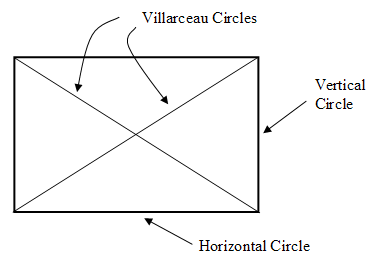Figure 2: Four circles on a torus passing through a point.

We know that RPM algorithm simulates a multi-particle dynamic system confined on the flat torus surface. For some unknown reasons, RPM maps often form symmetry patterns alone the diagonal lines, e.g. along the Villarcea circles. For instance, when we map the sphere to the tours, RPM algorithm cuts the sphere into two equal sized half and map them as two discs to the torus surface. The two discs are positioned alone a a diagonal line as depicted in the following picture:Figure 3: RPM Map of a sphere

We see in above picture that only one disc is completely displayed as a whole, the other one has been cut by the edges into 3 or 4 pices (which will form a complete disc when we glue the opposite edge together). The whole picture is more or less symmetric with respect to the left-up-to-right-bottom diagonal line. We can get a better visualization of above image when we tile multiple copies of above map together as be show in the following picture:Figure 4: Tiling duplicates of Figure 3.

Now, it would be helpful for the purpose of visualization if we can show the two discs as whole discs in a single map without using the tiling techniques. We can achieve this by transforming the coordinator from the two vertical and horizontal edges to the two diagonals (i.e. the two Villarceau circles). The transformation is schematically depicted in the following diagram: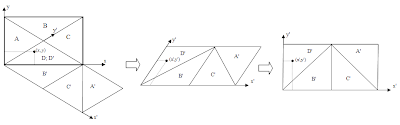Figure 5: Rotation to the diagonal circles.

We call the above transformation the diagonal rotation as it basically rotate the coordinator to the diagonals. Formally, the diagonal rotation is done by the following forms:In above forms w and h are the width and height of the fundamental rectangle respectively. d is the length of its diagonal. The above forms transforms points from the region D to D'. For points from other regions, we have to apply some simple shifting after the transformation as indicated in Figure 5.

As indicated in Figure 5, the two diagonals will become the edges of the transformed map. Thus, in order to avoid the resulting map be cut off by the edges we should shift a RPM map away from the diagonals through torus cyclical shifting. For our sphere example we can shift the map, for instance, to the following position:Figure 6: Sphere map shifted off from the diagonal lines.

When we now apply the diagonal rotation on the map in Figure 6 we will get the following rotated map:Figure 7: The sphere map after diagonal rotation.

Comparing with the original RPM map in Figure 3, the rotated map in figure 7 is obviously easier to investigate for human eyes.

It should be pointed out that a RPM map after diagonal rotation is no long a torus map in the sense that you cannot tile the map to fill 2D space the same way as be shown in Figure 4. Since this will break the edge-connectivity as indicated in Figure 5. But you can fill the 2D space with the rotated RPM map like building a bridge wall: you tile the map site by site to build a continuous lay, then build next lay the same while shift the lay to the left (or right) for half block. The following picture depicts this characteristics:Figure 8: Tiling with rotated map.

## Friday, April 4, 2008

### The Equivalence of Cooling and Expanding Dynamics

The RPM (relational perspective map) algorithm has a seemly add-hoc measure that gradually decreases the learning rate to force the convergence of the algorithm. I have tried to avoid such measure with various methods (e.g. conjugated algorithm exploring second order gradient), but was not able to find anything working.

I have notice a significant problem here: In the original Newton-Raphson algorithm, the algorithm should converge to a local minimum if the learning rate is sufficiently small. But, this not so for the optimization problem of RPM. The algorithm never converges, no matter how small the learning rate is (in a way that is doable with double precision arithmetic). If I keep the learning rate constant at certain small value, the simulated dynamic system goes in to an equilibrium state with constant variance.

Thus, it looks like NR method, as it is, does not work for massively complex dynamic system, it is not stable enough. Letting the learning rate gradually vanish seems to be the simplest method to enforce the convergence.

Mathematically, a very fine granulated gradient descent algorithm should be able to find a local minimum. But, I think this is practically not possible. To understand this, we notice that the learning rate practically controls the average variance of the positions of the simulated particles. That means, the learning rate is a kind of "temperature" of the system. In physics, we know that if we keep the temperature constant, the system will never converge to a frozen state. We can only reach that state when we gradually reduce the temperature to zero.

It is interesting to notice that the cooling dynamic system simulated by RPM is theoretically equivalent to a n-body dynamic system that is ever expanding. To see this, we notice that the learning rate is directly linked to the average variance of the particle's positions. Thus, instead of gradually lowering learning rate, we can just reduce the scale of unit length of the space compared to total size of the space (e.g. the size of the torus). The latter is again equivalent to a system in which we keep the scale of unit length constant, but gradually expand the size of the whole space.

We see here certain similarity of RPM model with the inflationary big-bang model from cosmology. Whereas RPM keeps the total size of the system stationary and gradually reduces the energy, the big-bang model keeps the total energy constant but let the universe expands. It is probably not a too crazy idea to consider a dual big-bang model that keeps the apparent universe stationary, but magically reduces the total energy/mass/temperature.

## Monday, March 10, 2008

### The "Big Three" Data Categories

Data analysis is an essential part of science and engineering. As a guide to deal with various data analysis tasks, I find it helpful to group data into three basic categories:
• Multidimensional tabular data (e.g . tables of numbers and text).
• Time series data (e.g. image, audio/video data).
• Graphics oriented data (e.g. tree and network structured data).
All data analysis methods can be grouped in to three categories depending on which data category they target. So, for instance, wavelet transformation is a method for time series; whereas MDS are methods for multidimensional data. Context free grammar and text parsers are mainly tools for graphics oriented data.

Furthermore, all software applications can also be grouped in to three corresponding categories. A software package that supports multiple data categories (like java system, Matlab) is either a generic tool that requires further software for end-users; or it is hopelessly difficult to design, implement, understand and to use.

When I encounter a new data analysis problem I first identify its category, then I start to search for methods and software packages targeting that category. I would not try, for instance, to use MDS or SQL to analyze images or video/audio data.

## Thursday, February 21, 2008

### VisuMap 2.6 Released

We have released VisuMap 2.6 today. This release includes some significant internal architecture change that may simplify future development.

We have considered implementing DirectX based code with WPF or XNA, but both libraries have turned out to be inadequate for our purpose. WPF does not support sprite; whereas XNA requires shader 1.1 as a minimum that is too much for our customers.

Apart from many accumulative improvements we have implemented a new data view, called mountain view, for high dimensional data. This feature can be considered as 3D extension of the existing value diagram. Mountain view also uses DirectX for fast navigation. The following is snapshot of the mountain view window:## Tuesday, January 8, 2008

### P. Dirac about geometric and algebraic way.

Recently, during my search for information about projective space I bumped into an interesting script of P. Dirac with title "Projective Geometry, Origin of Quantum Equations".
The script is made from a talk Dirac gave in 1972. He seemed to talk to a general public, so the talk was rather inform.

In his talk Dirac briefly described projective geometry and argued that projective geometry is more appropriate than Euclidean geometry as a mathematical structure for quantum theory. I was not able to really understand the link between projective geometry and quantum theory, but I believe his view was fundamentally correct in theoretical physics.

What has interested me the most was his philosophical comments about geometry and algebra. In mathematical works you will, according to Dirac, either prefer the algebraic way or the geometrical way. The algebraical way is more deductive where you start with equations or axioms and follow rules to get to interesting results. The geometrical way is more inductive where you start with some concrete pictures and try to find relationship among the pictures.

Dirac put himself into the geometrical camp. But he lamented that his works rather appear more algebraic (e.g. lots of equations) although he used a lot of geometrical methods. The reason for this is that it was pretty awkward to produce and print pictures in published papers. Writing equations was just much easier for him (and probably most other theorists in his time) than drawing pictures. So, it was rather a technique limit that led to heavy algebraic appearance of his works.

That technique limit seems to disappear with the availability of modern computers. I am wandering what impact could it have had if the modern computers were available to those great scientists. On the other hand, in the current academic world the algebraic way still seems to dominate the stage: a paper with a lot of differential equations would be considered more scientific than a paper with a lot pictures.

The mission statement of VisuMap Technologies is unleashing human visualization power for complex high dimensional data. It is indeed our grandiose ambition to revive visualization as first ranking tool to do science, that has somehow lost its glory since Rene Descartes invented his coordinate system. In order to achieve this we not only need new software, new methodologies and theories, but also people who embrace visual way to do scientific research. So, there is still a long way to go!

I feel pleased that Euclidean space has been considered inadequate by Dirac for quantum theory. Since I have felt for long time that the open Euclidean space is not appropriate for generic study of high dimensional data. For instance, some concepts like left/right, centers/peripherals (which make sense in Euclidean space) can not be applied intuitively to high dimensional data. For this reason, I have called RPM (relational perspective map) as MDS on closed manifolds. RPM basically simulates a non-stationary dynamic system on a closed manifold. Interestingly, modern physics offered some useful tools to do that. I have looked in to several low dimensional manifolds as our image space (like sphere, torus and real projective space). Our long term plan is to evolve the image space to more expressive structures to visualize high dimensional data.